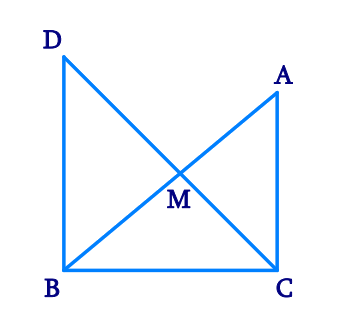In the verge of coronavirus pandemic, we are providing FREE access to our entire Online Curriculum to ensure Learning Doesn't STOP!

# Ex.7.1 Q8 Triangles Solution - NCERT Maths Class 9

Go back to  'Ex.7.1'

## Question

In right triangle $$ABC$$, right angled at $$C$$, $$M$$ is the mid-point of hypotenuse $$AB$$. $$C$$ is joined to $$M$$ and produced to a point $$D$$ such that $$DM = CM$$. Point $$D$$ is joined to point $$B$$ (see the given figure).

Show that:

(i) $$\Delta AMC \cong \Delta BMD$$

(ii) $$\angle DBC$$ is a right angle.

(iii) $$\Delta DBC \cong \Delta ACB$$

(iv) \begin{align}CM = \frac {1} {2}AB \end{align}

Video Solution
Triangles
Ex 7.1 | Question 8

## Text Solution

What is Known?

$$M$$ is the mid-point of hypotenuse $$AB,$$

$$\angle C = 90^\circ$$ and $$DM = CM$$

To prove:

(i) $$\Delta AMC\cong \Delta BMD$$

(ii) $$\angle DBC$$ is a right angle.

(iii) $$\Delta DBC\cong \Delta ACB$$

(iv) $$CM=\frac{1}{2}AB$$

Reasoning:

We can show two triangles AMC and BMD congruent by using SAS congruency rule and then we can say corresponding parts of congruent triangles will be equal means angle ACM will equal to angle BDM, which are alternate interiors angle and can conclude DB is parallel to AC. Now it will help to find angle DBC by co-interior angles. Similarly triangles DBC and ACB will be congruent by using SAS criterion and CM will be half of AB by using M mid-point.Steps:

(i) In $$\Delta AMC$$ and $$\Delta BMD,$$

\begin{align}&AM = BM\\& (\text{M is the} \text{ mid-point of AB})\\\\&\angle AMC = \angle BMD\&\text{Vertically}\text{ opposite angles} )\\\\&\text{CM} = DM\left( \text{Given} \right)\\&\therefore \Delta AMC \cong \Delta BMD\\( &\text{By SAS}\text{ congruence rule})\\\\&\therefore AC = BD\left( \text{By CPCT} \right)\\&\text{And,}\,\angle ACM = \angle BDM\left( \text{By CPCT} \right)\end{align} (ii) \(\begin{align} \angle ACM\text{ }&=\angle BDM \end{align}

However, $$\angle ACM$$  and $$\angle BDM$$  are interior alternate angles.

Since alternate angles are equal,

It can be said that $$DB\, \|\,AC$$

\begin{align} \angle DBC+\angle ACB&={180}^{\circ}\\text{Co-interior}&\text{ angles }) \\\\ \angle DBC+{90}^{\circ}&={180}^{\circ} \\ \therefore \angle DBC&={90}^{\circ} \\ \end{align} (iii) \(\Delta DBC\,\text{ and}\,\Delta ACB

\begin{align}\\DB&=AC\;(\text{Already proved})\\\angle DBC&=\angle ACB\\text{Each }&\, \text{angle measures }90^{\circ})\\\\BC&=CB\;(\text{Common})\\\therefore \Delta DBC & \cong \Delta ACB\\(\text{SAS }&\text{congruence rule})\\\end{align} (iv) \( \Delta DBC\cong \Delta ACB

\begin{align}\\AB&=DC \;(\text{By }CPCT)\\AB&=2\,\text CM \\ \therefore CM&=\frac{1}{2}AB\\\end{align}

Video Solution
Triangles
Ex 7.1 | Question 8

Learn from the best math teachers and top your exams

• Live one on one classroom and doubt clearing
• Practice worksheets in and after class for conceptual clarity
• Personalized curriculum to keep up with school